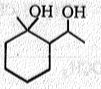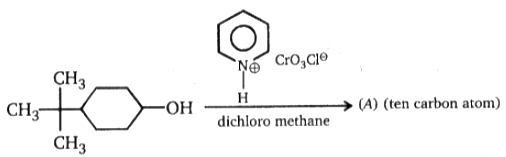1.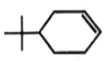2.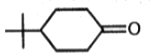3.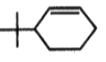4.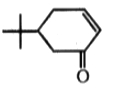Concept Questions :-

Alcohols: Preparation, Physical and Chemical Properties
High Yielding Test Series + Question Bank - NEET 2020

Difficulty Level: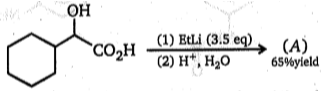; Chemist added extra 0.5 mole of Et-Li in above reaction to obtain product (A), which is ?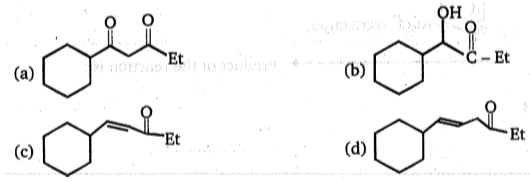Concept Questions :-

Alcohols: Preparation, Physical and Chemical Properties
High Yielding Test Series + Question Bank - NEET 2020

Difficulty Level: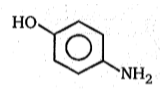$\underset{100°\mathrm{C}}{\overset{{\mathrm{AC}}_{2}\mathrm{O}}{\to }}$(A); Product (A) of reaction is:

(a)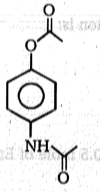(b)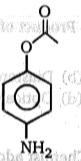(c)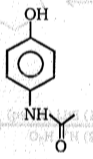(d)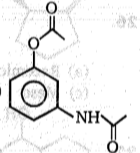Concept Questions :-

Alcohols: Preparation, Physical and Chemical Properties
High Yielding Test Series + Question Bank - NEET 2020

Difficulty Level:

Which is the best reagent to convert isopropyl alcohol to isopropyl bromide ?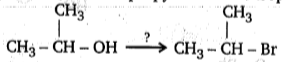(a) HBr
(b) SOBr2
(c) Br2
(d) CH3MgBr

Concept Questions :-

Mechanism of Dehydration, Uses, Methanol and Ethanol
High Yielding Test Series + Question Bank - NEET 2020

Difficulty Level: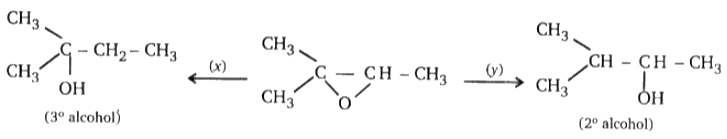Find missing reagents.
1. x = LiAlH4, y = NaBH4

2. x = LiAIH/ AlCl3, y = LiAlH4

3. x = LiAIH4, Y = LiAIH/ AlCl3

4. x = H/ Ni, y = H2 / Pt

High Yielding Test Series + Question Bank - NEET 2020

Difficulty Level:

Which are not cleaved by HIO4?
I : glycerol
II : glycol
III: 1, 3-propanediol
IV: methoxy-2-propanol

1. I, II, III, IV

2. I, II

3. II, III

4. III, IV

Concept Questions :-

Alcohols: Preparation, Physical and Chemical Properties
High Yielding Test Series + Question Bank - NEET 2020

Difficulty Level:

Which of the following compounds are not oxidized by HIO4?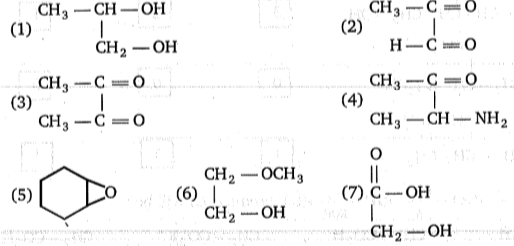(a) 5,6,7     (b) 4,5,6,7     (c) 6,7     (d) 3,4,5,6,7

Concept Questions :-

Ethers: Preparation, Physical & Chemical Properties, Uses
High Yielding Test Series + Question Bank - NEET 2020

Difficulty Level:

Succinic acid $\stackrel{∆}{\to }$(A)$\underset{∆}{\overset{{\mathrm{NH}}_{3}}{\to }}$(B)$\underset{\mathrm{KOH}}{\overset{{\mathrm{Br}}_{2}}{\to }}$(C); Product (C) will be:

(a) $\begin{array}{ccc}\underset{|}{{\mathrm{CH}}_{2}}& -& {\mathrm{CO}}_{2}\mathrm{H}\\ {\mathrm{CH}}_{2}& -& \begin{array}{cc}{\mathrm{CH}}_{2}& \begin{array}{cc}-& {\mathrm{NH}}_{2}\end{array}\end{array}\end{array}$

(b) $\begin{array}{ccc}\underset{|}{{\mathrm{CH}}_{2}}& -& {\mathrm{CO}}_{2}\mathrm{H}\\ {\mathrm{CH}}_{2}& -& {\mathrm{NH}}_{2}\end{array}$

(c) $\begin{array}{ccc}\underset{|}{{\mathrm{CH}}_{2}}& -& {{\mathrm{CO}}^{-}}_{2}{\mathrm{K}}^{+}\\ {\mathrm{CH}}_{2}& -& {\mathrm{NH}}_{2}\end{array}$

(d) $\begin{array}{ccc}\underset{|}{{\mathrm{CH}}_{2}}& -& {\mathrm{CO}}_{2}\mathrm{H}\\ {\mathrm{CH}}_{2}& -& \begin{array}{cc}{\mathrm{CH}}_{2}& \begin{array}{cc}-& \mathrm{Br}\end{array}\end{array}\end{array}$

Concept Questions :-

Alcohols: Preparation, Physical and Chemical Properties
High Yielding Test Series + Question Bank - NEET 2020

Difficulty Level: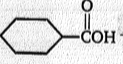L$\stackrel{{\mathrm{CH}}_{3}\mathrm{Li}}{\to }$M$\stackrel{{\mathrm{H}}_{3}{\mathrm{O}}^{+}}{\to }$ N + CH3OH

Product (N) is: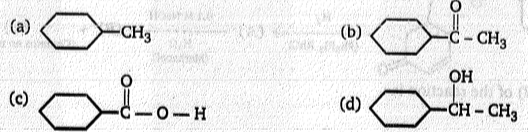Concept Questions :-

Alcohols: Preparation, Physical and Chemical Properties
High Yielding Test Series + Question Bank - NEET 2020

Difficulty Level: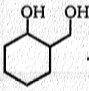$\stackrel{\mathrm{PCC}}{\to }$(A)$\underset{{\mathrm{H}}^{+}}{\overset{\mathrm{OH}-\mathrm{CH}2-\mathrm{CH}2-\mathrm{OH}}{\to }}$(B)(C)$\stackrel{{\mathrm{NaBH}}_{4}\mathrm{EtOH}}{\to }$(D) Product (D) in above reaction Is:

(a)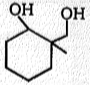(b)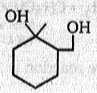(c)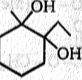(d)